•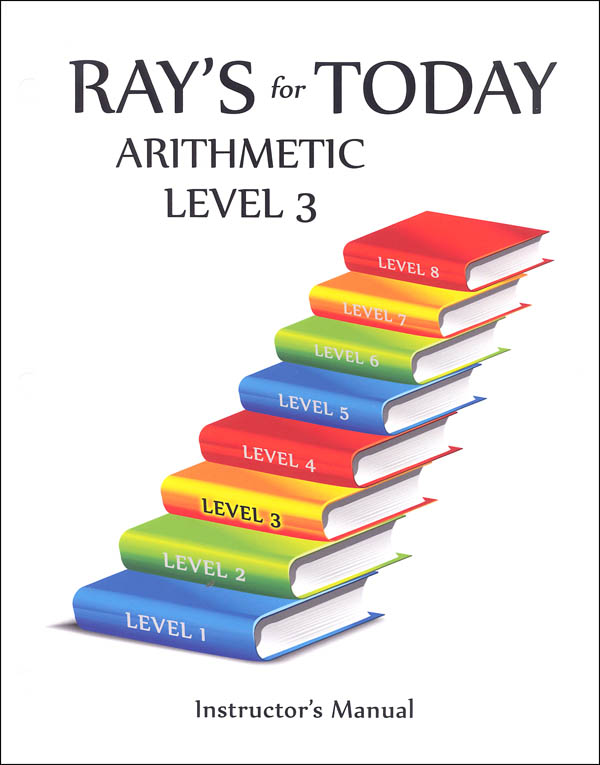•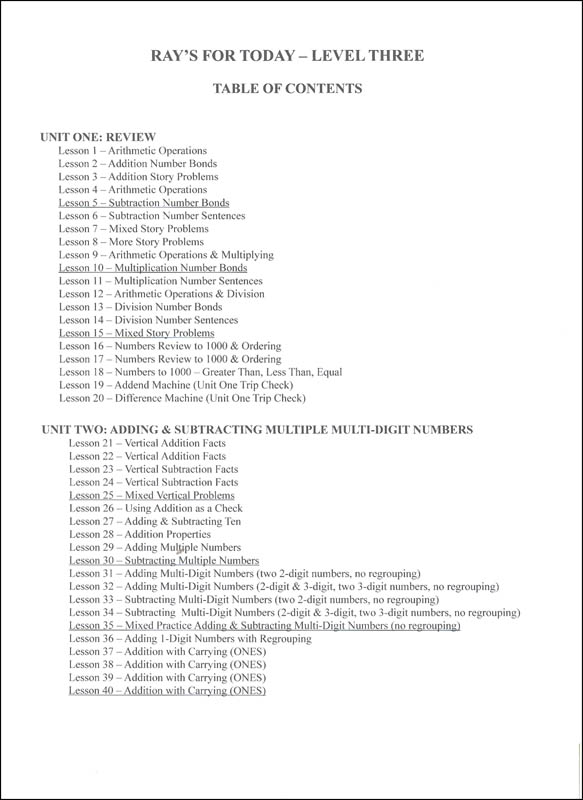•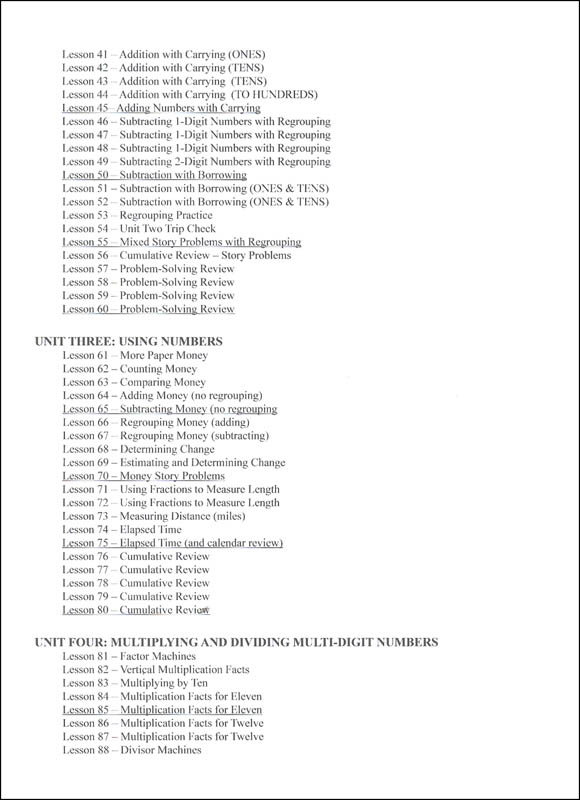•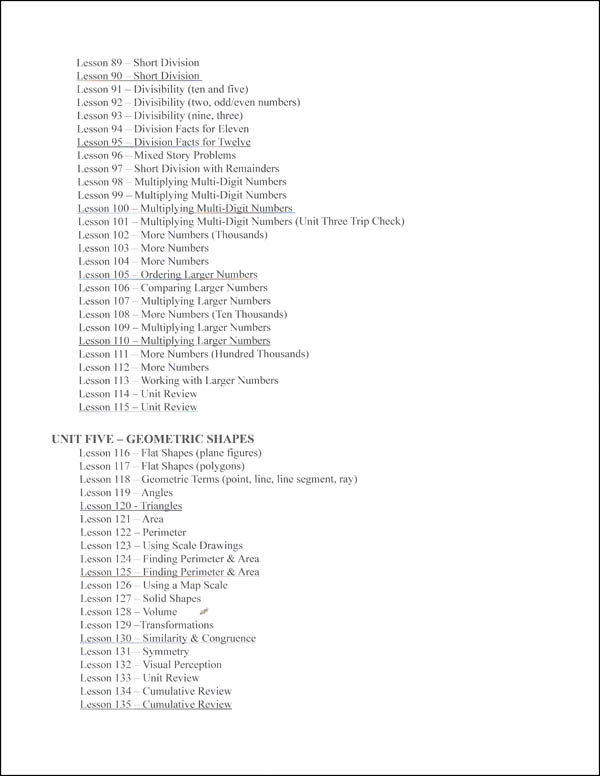•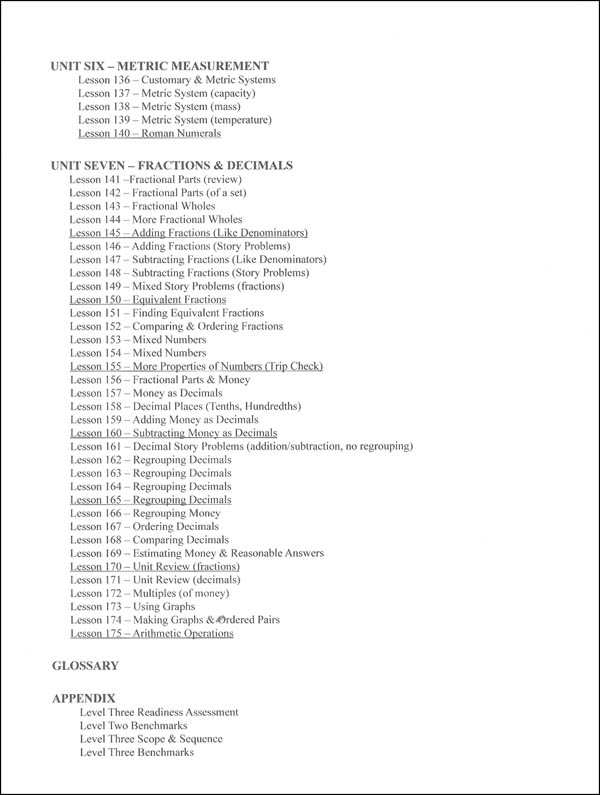•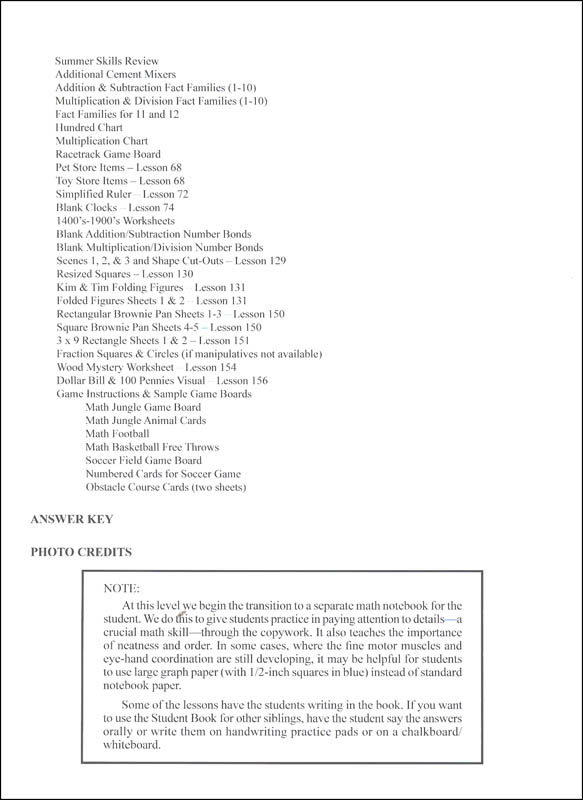•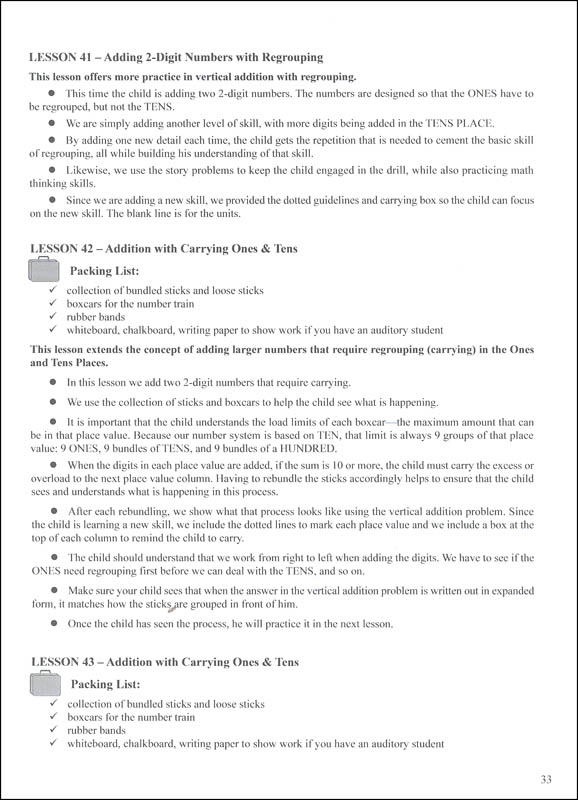•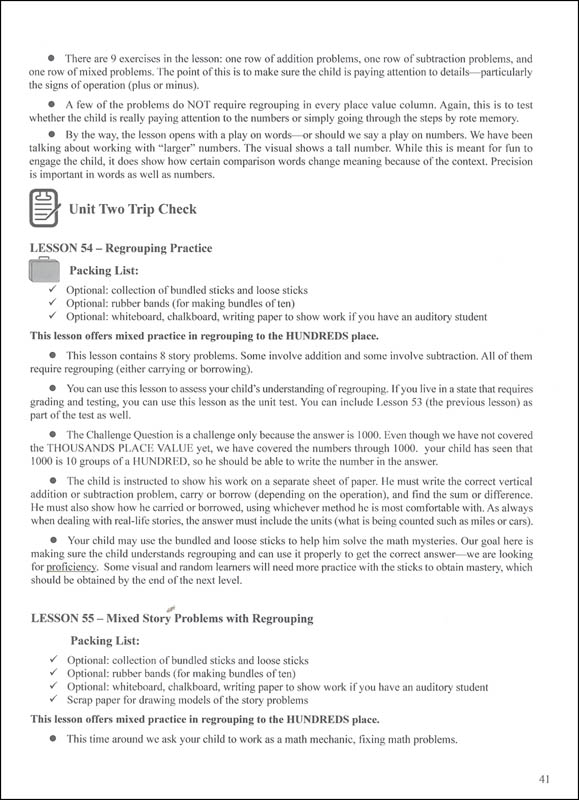•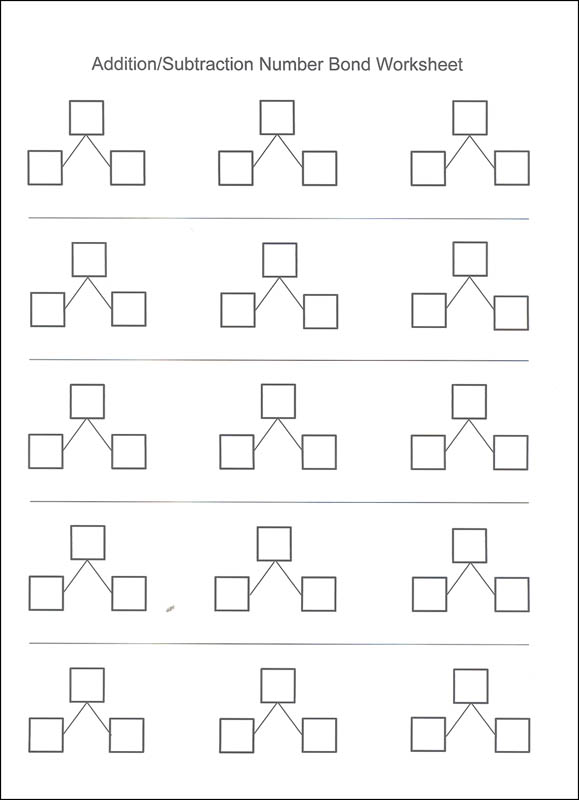•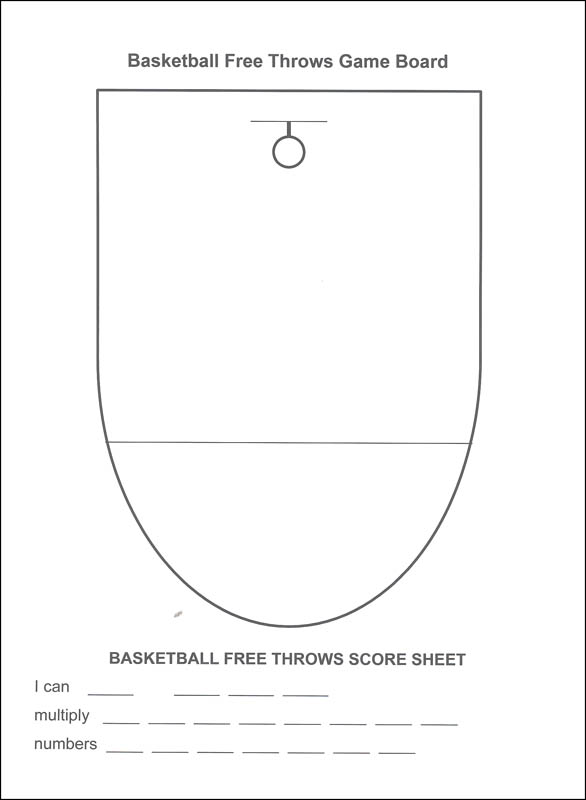•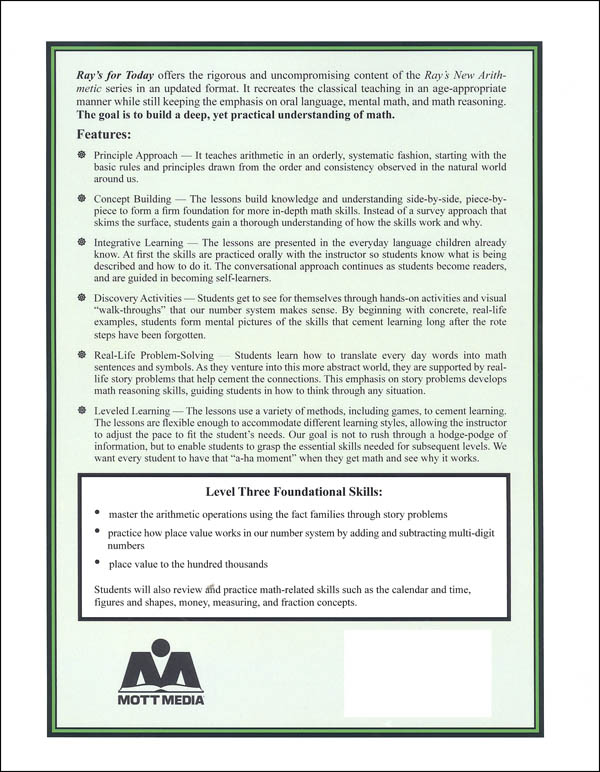# Ray's for Today Level 3 Instructor's Manual

# 069638

Our Price: \$29.50
Retail: \$29.99
Save: 1.63% (\$0.49)
In Stock.
Qty:
Qty:

Item #: 069638 9780880620888 3-4

#### Publisher Description:

Level 3
The essential learning objectives are:
• master the arithmetic operations using the fact families through 10 using story problems and number problems
• practice how place value works in our number system by adding and subtracting multi-digit numbers
• learn place value to the hundred thousands
Category Description for Ray's for Today Level 3:

Level 3 for ages 8 and 9 includes number concepts; arithmetic operations of addition, subtraction, multiplication, short division, introduction to order of operations; money; time; measurement including fractions and metric; geometric constructions; fractions, including comparing and ordering fractions and addition and subtraction with like denominators; decimals, including ordering and comparing, decimals in money, and place value to the hundredths place; graphing, circle and line graphs, using a grid and ordered pairs; problem-solving with multi-step word problems, estimating, and estimating. The goal for level 3 is that the student master arithmetic operations using the fact families through 10 using story problems and number problems, practice how place value works in our number system by adding and subtracting multi-digit numbers, as well as to learn place value to the hundred-thousands.

Category Description for Ray's for Today:

If you want to be sure that you are covering good, solid math, Rays for Today puts the methodology and content of Rays Arithmetic into a format for today’s homeschool families. Rays for Today doesn’t look anything like the little brown, hardcover books of Rays Arithmetic, but almost all the problems from the original texts are included. Rays employs a concrete, to pictorial, to conceptual presentation of math, progressively builds on basic math concepts, and provides good mental and oral math opportunities. For those frustrated with the new math programs that are on the market today, Rays for Today offers them a solution.

The original Rays was used in a group setting, so the textbooks didn't include the teaching information; it was intended for the teacher to present the information, but Rays for Today has added the lesson/teaching information right in the student text. There are 170 lessons in each of Level 1 and 2, and 175 lessons in levels 3 and 4 of the series. Both the Student Text and Instructors Manual are 3-hole punched for you to place them in your own binders.

The Student Text is easy to use, and students are provided space on each page to work out the lesson. Instruction is presented, examples provided, then an exercise. Books are printed in black and white with no fancy graphics or pictures. The only illustrations you see directly support the lesson or exercises.

The Instructors Manual explains how the program works, clarifies your role as a parent/teacher, lists suggested manipulatives, and outlines how to assess. You can use the suggested manipulatives or household items, which are: more than 100 counters, chalkboard/whiteboard or blank paper (your choice), jumbo-sized craft sticks, rubber bands, handwriting practice pads (optional), 12-month calendar, 100 pennies, 10 dimes, 4 quarters, 2 half dollars, one dollar bill, crayons, 2-4 treats to sell, 5 game markers, paperclip, various sized toys, plastic glasses, plastic fork, measuring cups, empty gallon jug, funnel, various templates found at the back of the Instructors Manual. These are some of the items used in both Levels 1 and 2, but a few other items are listed at the beginning of each unit. There are no formal assessments, but there are suggestions to help you out. Cement Mixers are oral drills that are found at the beginning of each unit (three units in Level 1 and five units in Level 2). These are used to cement the facts into the minds of young learners. Each unit begins with an overview, suggested schedule, background, goals, materials list, and extension activities. Each lesson within the unit includes materials needed and step-by-step instruction for teaching the concept that is very easy to follow. The appendix includes a scope and sequence for that level, end-of-year benchmark checklist, summer skills review, cement mixers checklist, templates to be copied for the lessons, and an answer key.

Rays Arithmetic isn't one level per grade, and neither is Rays for Today. You can begin Level 1 at grade one or two and progress from there.

Level 1 includes reading, writing, and counting through 100, shapes, one more, one less, grouping by ten, calendar, addition with up to three numbers, number bonds, story problems, money, time, temperature, and length.

Level 2 includes reading, writing, counting, and conceptually understanding numbers through 1,000, multiplication of numbers with products up to 100 and dividing numbers with dividends up to 100, division fact families 1-10, place value to thousands, graphs, weight, capacity, shapes, story problems, perimeter, and skip counting by 10s and 100s.

Level 3 for ages 8 and 9 includes number concepts; arithmetic operations of addition, subtraction, multiplication, short division, introduction to order of operations; money; time; measurement including fractions and metric; geometric constructions; fractions, including comparing and ordering fractions and addition and subtraction with like denominators; decimals, including ordering and comparing, decimals in money, and place value to the hundredths place; graphing, circle and line graphs, using a grid and ordered pairs; problem-solving with multi-step word problems, estimating, and estimating. The goal for level 3 is that the student master arithmetic operations using the fact families through 10 using story problems and number problems, practice how place value works in our number system by adding and subtracting multi-digit numbers, as well as to learn place value to the hundred-thousands.

Level 4 is for ages 9 and 10 and covers number concepts that include whole and rational numbers, fractions, decimals, mixed numbers, and zero, commutative, identity, and associative properties for addition and multiplication, powers of ten, rounding, estimating; arithmetic operations that include vocabulary for the four functions, signs of operations, mental math, functions to millions, multiplying 2-digit numbers, long division, and dividing by 2-digit numbers; money; time; measurement includes customary measure and abbreviations, metric measure and abbreviations; geometric constructions review of plane and solid figures, regular and irregular figures, triangles and angles, circles, area, perimeter, point, lines, rays, protractor, diagonals, and tangrams; fractions includes wholes, proper and improper fractions, mixed numbers, addition and subtraction with unlike denominators, and reducing; decimals in money, place value through thousandths, equivalent decimals, comparing and ordering, and addition and subtraction of decimals; graphing averages, median and mode; problem-solving; Venn Diagrams. The goal of level 4 is that students become proficient at adding and subtracting fractions and mixed numbers, recognize and work with numbers through the millions, multiply by a 2-digit number, be able to do long division by 1-digit and 2-digit numbers, be introduced to decimals and decimal place values.

Level 5 is for ages 10-11 and includes number concepts, arithmetic operations up through long division, money, time, measurement, geometric constructions, fractions, decimals, percents, ratios graphing & statistics, and problem-solving. The objectives for Level 5 are that students become proficient at recognizing and working with numbers through billions, multiplying and dividing by a multi-digit number, use all four operations with fractions and mixed numbers, decimal concepts, factoring and related concepts, and be introduced to percents and ratios.

Level 6 is for ages 11-12 and includes the same areas of math work as that of Level 5 but goes deeper. The objectives for Level 6 are that students master recognizing and working with numbers through trillions, math facts and all four arithmetic operations with whole numbers. Students should become proficient at unit conversions in measurement and time, all four operations with fractions and decimals, percent concepts and finding the percent of a number, recognizing the relationships between fractions, decimals, and percents, and converting between the three, solving problems based on A=B of C, powers of ten, working with integers and ratios, and begin learning about cancellation and exponents.

The topics mentioned for each level are just some of the major topics covered - this is definitely a comprehensive math program.

Levels 1 through 6 are now available from this eight-level program. Intermediate Math - Level 7 for ages 12 &13 is planned for release in 2020 and Pre-Algebra - Level 8 for ages 13 &14 is planned for 2021.

If you want that old school feel to your math program, straight-forward and uncomplicated, give Rays for Today a try. It’s easier to use than the original Rays Arithmetic, but still provides the same solid math foundation as the original.

Category Description for COMPREHENSIVE PROGRAMS - ELEMENTARY:

Primary Subject
Mathematics
3
4
ISBN
9780880620888
Format
Paperback
Brand Name
Mott Media
Weight
1.65 (lbs.)
Dimensions
11.0" x 8.5" x 0.62"